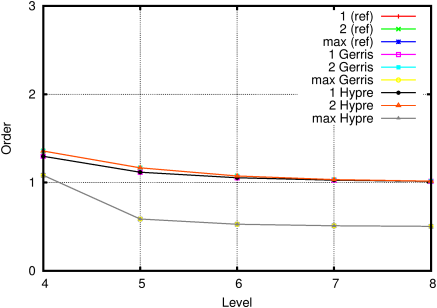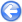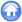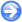### 2.2PASS: Convergence of the Poisson solver with solid boundaries

Author
Stéphane Popinet
Command
sh circle.sh circle.gfs
Version
100325
Required files
circle.sh res-7.ref error.ref order.ref solution.gfv
Running time
3 minutes 51 seconds

Another of the test cases presented in Popinet . A circular solid boundary of radius 0.25 is embedded in the domain. The same right-hand-side is used.

This time the problem does not have an analytical solution and we use Richardson extrapolation to estimate the error for a given resolution.

 Figure 13: Solution of the Poisson equation.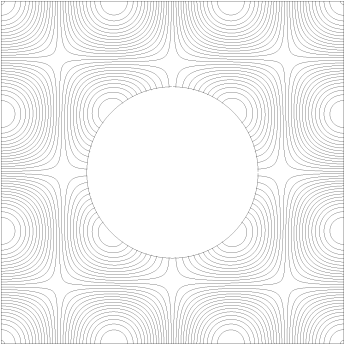Figure 14: Evolution of the residual.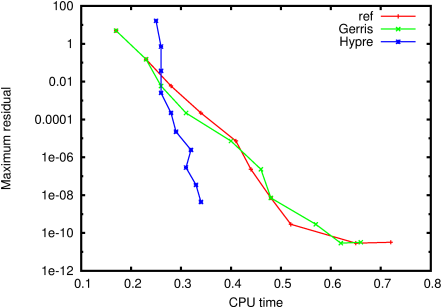Figure 15: Average reduction factor.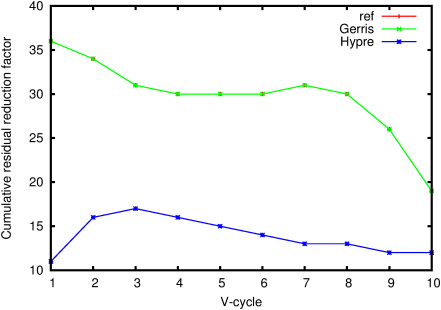Figure 16: Evolution of the error as a function of resolution.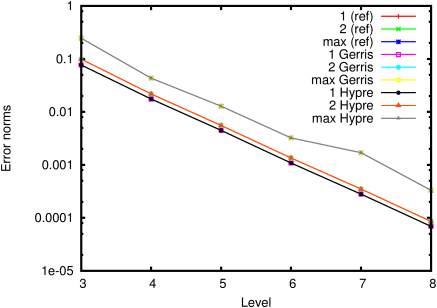Figure 17: Corresponding convergence order.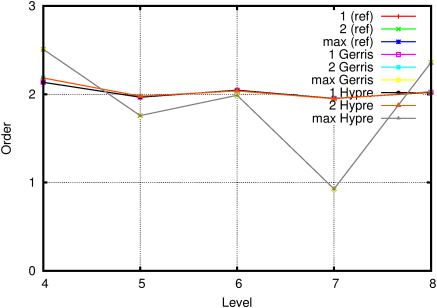#### 2.2.1PASS: Star-shaped solid boundary

Author
Stéphane Popinet
Command
sh ../circle.sh star.gfs
Version
100325
Required files
res-7.ref error.ref order.ref solution.gfv
Running time
3 minutes 39 seconds

A similar but more difficult test using a star-shaped solid boundary defined in polar coordinates as

 r(θ)=0.2765+0.079cos(6θ)

The convergence rate is smaller because the shape of the boundary is not represented properly on the coarsest levels of the multigrid hierarchy.

 Figure 18: Solution of the Poisson equation.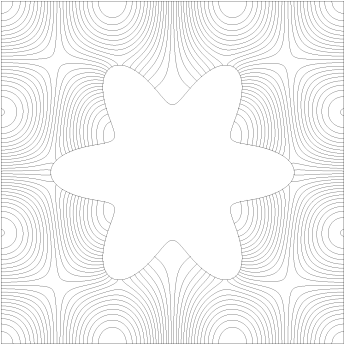Figure 19: Evolution of the residual.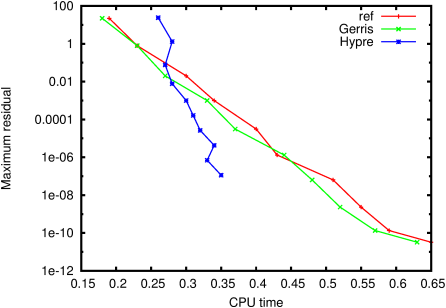Figure 20: Average reduction factor.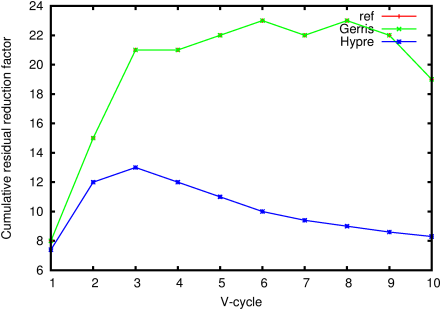Figure 21: Evolution of the error as a function of resolution.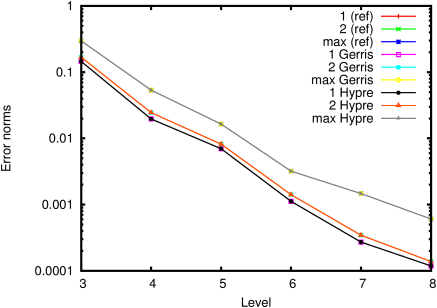Figure 22: Corresponding convergence order.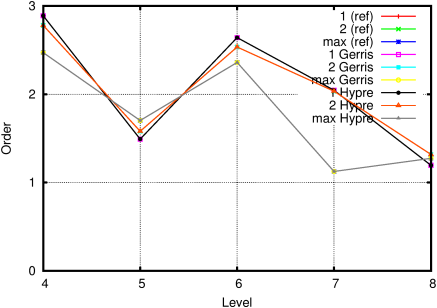#### 2.2.2PASS: Star-shaped solid boundary with refinement

Author
Stéphane Popinet
Command
sh ../circle.sh refined.gfs
Version
100325
Required files
res-7.ref error.ref order.ref solution.gfv
Running time
5 minutes 33 seconds

Same as the star test but with two levels of refinement added near the solid boundary.

 Figure 23: Solution of the Poisson equation.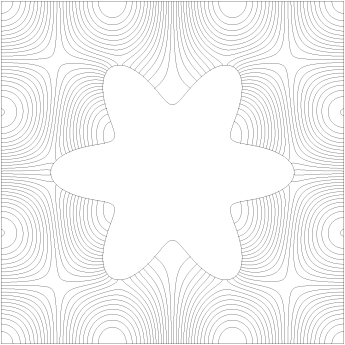Figure 24: Evolution of the residual.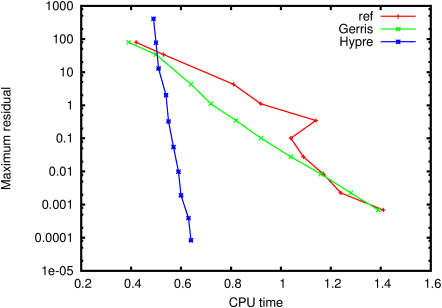Figure 25: Average reduction factor.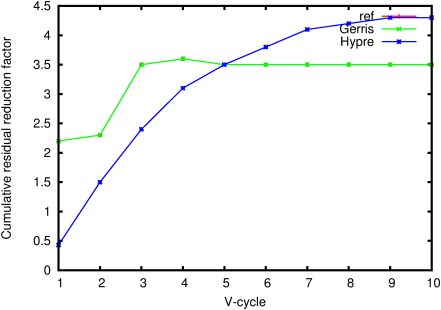Figure 26: Evolution of the error as a function of resolution.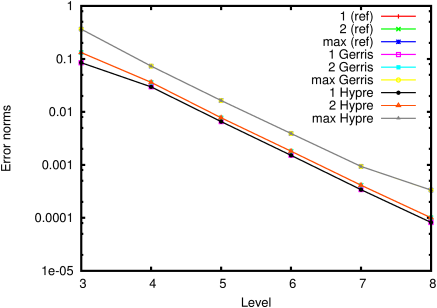Figure 27: Corresponding convergence order.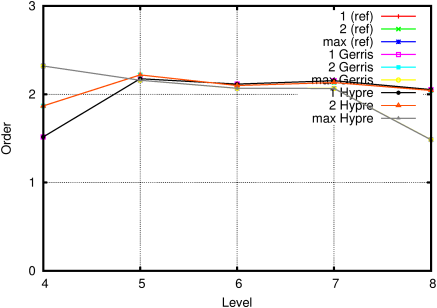#### 2.2.3PASS: Thin wall at box boundary

Author
Stéphane Popinet
Command
sh ../circle.sh thin.gfs
Version
100325
Required files
res-7.ref error.ref order.ref solution.gfv
Running time
14 minutes 40 seconds

Boxes are used to setup a similar problem but with an infinitely thin wall.

 Figure 28: Solution of the Poisson equation.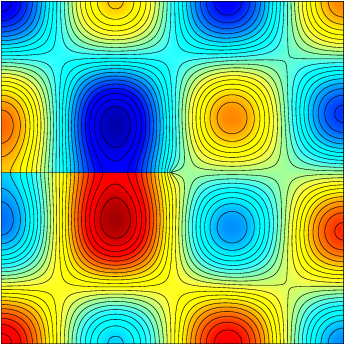Figure 29: Evolution of the residual.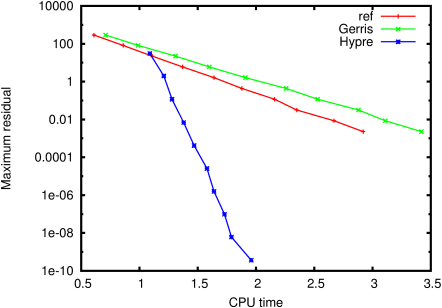Figure 30: Average reduction factor.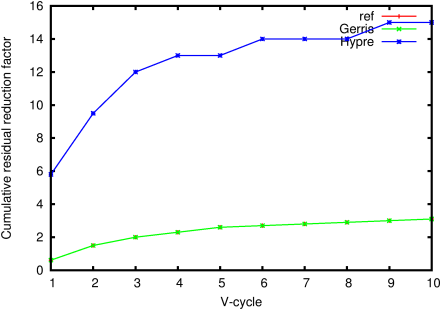Figure 31: Evolution of the error as a function of resolution.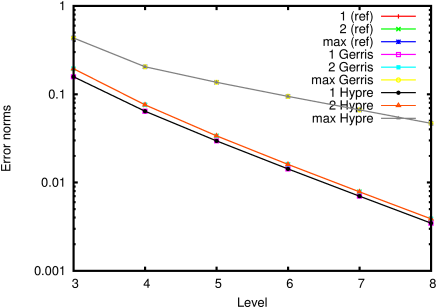Figure 32: Corresponding convergence order.